# Cochain

(diff) ← Older revision | Latest revision (diff) | Newer revision → (diff)

A homogeneous element of an Abelian cochain group(or, in the general case, a module), i.e. a graded Abelian group equipped with an endomorphismof degreesuch that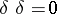. The endomorphismis called the coboundary mapping or the coboundary.

A cochain group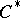usually arises as a groupor, whereis an arbitrary Abelian group, called the coefficient group, and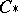is a chain group, i.e. a graded Abelian group equipped with an endomorphism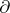of degree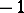(the boundary mapping or boundary) with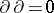. In this situation the mappingon the group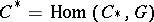is defined as the adjoint of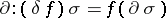, where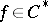,.

Given a topological space, one defines the group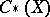of singular chains as the Abelian group of formal finite sums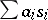, where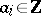and theare arbitrary singular simplices in, i.e. continuous mappings of the standard simplex into. A singular cochain inwith coefficients inis defined as a homogeneous element of the group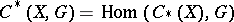.

Similarly, a simplicial-cochain of a simplicial complex inwith coefficients in an Abelian groupis defined as a homomorphism, whereis the group of-chains of, i.e. the group of formal finite sums, whereand theare-simplices in. In particular, a cochain in the sense of Aleksandrov–Čech in an arbitrary topological spaceis a cochain of the nerve of an open covering of.

Ifis a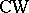-complex (and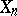denotes the-skeleton of), then the Abelian groupis called the group of-dimensional cellular cochains of the complex. The coboundary homomorphismis put equal to the connecting mappings of the triple.

In practice, the groupis frequently provided with an additional multiplicative structure, i.e. it is a graded algebra. In these cases the coboundary mappingpossesses the Leibniz property: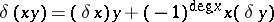, where the element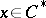is assumed to be homogeneous of degree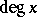. An example of such a graded cochain algebra is the algebra of differential forms on a smooth manifold, in which the exterior differential acts as coboundary.

How to Cite This Entry:
Cochain. Encyclopedia of Mathematics. URL: http://encyclopediaofmath.org/index.php?title=Cochain&oldid=17694
This article was adapted from an original article by A.F. Kharshiladze (originator), which appeared in Encyclopedia of Mathematics - ISBN 1402006098. See original article